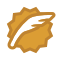# Weird Clock

My Tags (Edit)
 Source : ZJU Time limit : 3 sec Memory limit : 32 M

Submitted : 9417, Accepted : 1821

A weird clock marked from 0 to 59 has only a minute hand. It won't move until a special coin is thrown into its box. There are different kinds of coins as your options. However once you make your choice, you cannot use any other kind. There are infinite number of coins of each kind, each marked with a number d ( 0 <= 1000 ), meaning that this coin will make the minute hand move d times clockwise the current time. For example, if the current time is 45, and d = 2. Then the minute hand will move clockwise 90 minutes and will be pointing to 15.

Now you are given the initial time s ( 0 <= s <= 59 ) and the coin's type d. Write a program to find the minimum number of d-coins needed to turn the minute hand back to 0.

Input

There are several tests. Each test occupies a line containing two positive integers s and d.

The input is finished by a line containing 0 0.

Output

For each test print in a single line the minimum number of coins needed. If it is impossible to turn the hand back to 0, output "Impossible".

Sample Input

30 1
0 0

Sample Output

1
有必要说下的是这种标记方法 被访问的用 1 标记 ， 没访问的用 0 标记； 数数组去记录标记的位置
算法思想：
         第一步： 数据结构：要想到使用一个数组来标记 鈡所走的位置 1表示走过了 0 表示还没有走过
         第二步： ：算法求其余数 在一个while循环里面完成 知道余数为0 停止 见代码 while循环中 这里最关键
         第三遍 ：打印就可以了
源代码：
    WA 的不知道为什么 WA

#include<iostream>
#include<cstring>
using namespace std;

int main()
{
int a, b;
int c;
while(cin>>a>>b)
{
memset(c, 0, sizeof(c));
if(a == 0 && b == 0)
{
return 0;
}
if(a == 0)
{
cout<<"0"<<endl;
}
if(a != 0 && b == 0)
{
cout<<"Impossible"<<endl;
}
int t = 0;
while(a && c[a] == 0)
{//开始循环记录求余 记录指针停的位置
c[a] = 1;
a = a*(b+1)%60;
t++; // t 用来记录金币使用的个数
}
if(a != 0)
{
cout<<"Impossible"<<endl;
}
else
{
cout<<t<<endl;
}
}
return  0;
}


#include <stdio.h>

int main()
{
int s, p;

while(scanf("%d %d", &s, &p) == 2){
if((s == 0) && (p == 0)){
return 0;
}else if((s == 0) && (p != 0)){
printf("0\n");
}else if(s*(p+1) % 60 == 0){
printf("1\n");
}else if(s*(p+1)*(p+1) % 60 == 0){
printf("2\n");
}else{
printf("Impossible\n");
}
}

return 0;
}

wangxiaomingCSDN认证博客专家 架构 Spring Boot Redis

05-10219

#### HOJ 11104题解（简单DP）09-2951

#### HOJ_first_homework_F

06-21392

#### HOJ过200了

04-291900

#### 【ZOJ-4027】 Sequence Swapping(2018浙江省赛-D题）

08-08798

#### HDU - 1568 Fibonacci （斐波那契，大数取前几位

07-202961

#### C++ HOJ 火车进站

09-21186

#### Hoj2739

03-09553

#### HOJ Megaminx

02-18597

#### Hoj 2276 Count prime

07-23743

#### HOJ 1002题 题解©️2020 CSDN 皮肤主题: Age of Ai 设计师: meimeiellie点击重新获取扫码支付1.余额是钱包充值的虚拟货币，按照1:1的比例进行支付金额的抵扣。
2.余额无法直接购买下载，可以购买VIP、C币套餐、付费专栏及课程。余额充值